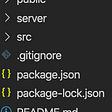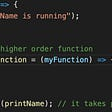# JavaScript — Return Object From Array

Let’s look at an exercise below to learn how to work with elements in an array and return a new object.

Q. Create `getData` function following the instructions below.

`**getData` function gets three parameters.**

`salesArr`: Amount of sales each day
ex)
`[["20190401", 34], ["20190402", 23], ["20190403", 29]]`

`reviewArr` : Amount of reviews each day
ex)
`[["20190328", 3], ["20190401", 0], ["20190403", 1]]`

`likeArr` : Amount of likes each day
ex)
`[["20190328", 98], ["20190401", 102], ["20190403", 125]]`

The above arrays are for examples only, which means the number of elements can be many more than 3.

Why change the arrays to objects? Because if we want to check the amount of sales, reviews, and likes for each day, we need to iterate each array, which means the data structure is not convenient or efficient.

The returned object should have these following three properties.

`sumAmount` : Total Sales

`sumReview` : Total Reviews

`sumLike` : Total Likes

A. My solution is:

`function getData(salesArr,reviewArr,likeArr){ let sumData = function (arr) {let total = 0;for (let i = 0; i<arr.length; i++){ total += arr[i];} return total;}let result = { sumAmount: sumData(salesArr), sumReview: sumData(reviewArr), sumLike: sumData(likeArr)};return result;};`
1. First, I thought about what result we need to get when the function is executed. As the function gets three arrays as arguments, we can use the data of elements from those arrays and need to return a new object with the total number of sales, reviews and likes as its properties.
2. Let’s look at the structure of the arrays. Each array has each element as another array, and the array inside the array has elements of date, and number.
3. To get the total number of sales, reviews and properties, we know that we need to sum up the second element of each element array of the array:`[["20190401", 34], ["20190402", 23], ["20190403", 29]] // bolded numbers should be summed up.`
4. Because we need to do this for each element of the array, it looks like we need a function `sumData` with `for` loop:
`let sumData = function (arr) {let total = 0;for (let i = 0; i<arr.length; i++){ total += arr[i];} return total;}`

We can access the element mentioned in #3 by `arr[i]` (the second element in each element array). The variable `total` is initially declared as 0 which will be incremented as we iterate.

5.

`let result = { sumAmount: sumData(salesArr), sumReview: sumData(reviewArr), sumLike: sumData(likeArr)};`

Now we have the function to get the total number of sales, reviews and likes, let’s set them as properties of a new object `result` .

The property names (key) are written on the left of `:` . The property values (value) are on the right, which we can get by calling the function `sumData` for each array (ex. `sumData(salesArr)`).

--

--

--

## File Upload With React Hooks , Cloudinary And React-Dropzone## 10 Awesome Microsoft Visual Studio Extensions You Should Install## 11 Tools and Libraries for Testing in React## Scaling Frontend Applications — Coding Guidelines## Using React-Router and Hooks Router together for the Same Project## Web App as a Graph## Building CRUD Apis Using Express and Postgresql## Creating a Shopping Dapp with React.js and Thanos Wallet## 2D Laplace Equation with JavaScript## #0 Building a JavaScript & HTML browser RPG: Introduction## Reacting to ReactJS!## Explain Higher-Order Functions in javascript.